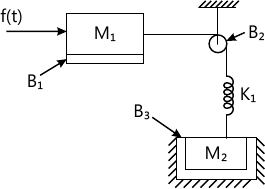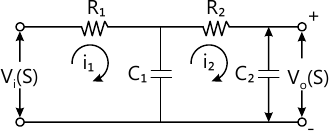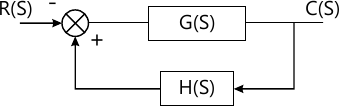Total marks: --
Total time: --
INSTRUCTIONS
(1) Assume appropriate data and state your reasons
(2) Marks are given to the right of every question
(3) Draw neat diagrams wherever necessary

1 (a) Define a control system. Explain with examples, open loop and closed loop control systems. List the merits and demerits of open loop and closed loop control system.
10 M
1 (b) For the mechanical system shown in Fig. Q1(b).
i) Draw the mechanical network
ii) Write the differential equations description the system.
iii) Draw the F-V analogous electrical circuit after writing the corresponding electrical equations.10 M

2 (a) For the circuit shown in Fig Q2(a). Draw the block diagram and determine the transfer function $\dfrac {V_p(S)}{V_1(S)}$ using block diagram rules.10 M
2 (b) For the system represented by the following equations, find the transfer function X(s)/U(s) by signal flow graph techniques
$x=x_1+\alpha_3 U \\ x_1=-\beta_1x_1+x_2+\alpha_2U \\ x_2=-\beta_2x_1+\alpha_1U$
10 M

3 (a) Explain the following time domain specification of a second order systems, with neat sketch i) Peak time ii) Delay time iii) Rise time iv) Maximum over shoot
v) Settling time.
6 M
3 (b) A system description by $\dfrac {d^2 y}{dt^2}+ \dfrac {8dy}{dt}+ 25y(t)=50x(t)$ Evaluate the response and maximum output for a step of 2.5 units.
8 M
3 (c) In the block shown in Fig. Q3(c) G(s)=A/S2 and H(s)=(ms+n). For A=10, determine the values of m and n for a step input with a time constant 0.1 sec; which give a peak over shoot of 30%.6 M

4 (a) What are the difficulties encountered while assessing Routh-Hurwitz criteria and how do you eliminate these difficulties, explain with examples.
6 M
4 (b) The open loop transfer function of a feedback control system is given by $G(s)H(s)= \dfrac {k}{S(s+4)(s^2+2s+2)}$
i) Using R-H criterion determine the range of K" for which the system will be stable
ii) If a zero at S=-4 is added to the forward transfer function
8 M
4 (c) Using R-H criterion, find the stability of a unity feedback system having closed loop transfer function $G(s)= \dfrac {e^{-s1}}{S(s+2)}$
6 M

5 (a) State the different rules for the construction of root locus.
8 M
5 (b) A feedback control system has open loop transfer function: $G(S)H(S)= \dfrac {k}{S(s+4)(s^2+4s+20)}$ Plot the root locus for K=0 to ? indicate all point on it.
12 M

6 (a) Explain co-relation between time domain and frequency domain for second order systems.
6 M
6 (b) The open loop transfer function of unity feedback control system is given by $G(s)H(s)= \dfrac {k}{s(1+0.00 ls)(1+0.25s)(1+0.1s)}$ Determine the value of K, so that the system will have a phase margin of 40°, what will be the gain margin. Use code plot.
14 M

7 (a) State and explain Nyquist stability criterion.
6 M
7 (b) Using Nyquist stability criterion, find the range of K for closed-loop stability $G(s)H(s)= \dfrac{K}{S(s^2+2s+2)}K>0$
14 M

8 (a) Explain properties and significance of state transition matrix.
10 M
8 (b) A linear time invariant system is characterized by the homogeneous state equation: $\begin{bmatrix} \dot{x_1} \\ \dot{x_2} \end{bmatrix} = \begin{bmatrix} 1 &0 \\1 &1 \end{bmatrix} \begin{bmatrix}x_1\\x_2\end{bmatrix}$ Compare the solution of homogeneous equation assume the initial state vector.
10 M

More question papers from Control Systems